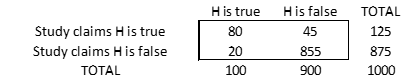The Briefing on last week’s Economist – Unreliable research – should ring a bell for regular readers of this blog.

The question is: What is the probability that a claim made by a piece of empirical research is in fact true?

This is the same as asking the probability that the child footballer coach is right, that homeopathic medicine works, or that you got a virus infection.

The corresponding table in the Economist example is:There are 1,000 studies, 100 of which are tests of true Hypotheses (BR=10%). Of these, 80 rightly claim that the hypothesis is true (TPR=80%) and 20 wrongly claim that it is false (FNR=20%). The rest of the studies test false hypotheses. Of these, 45 wrongly claim that the hypothesis is true (FPR=5%) and 855 rightly claim that it is false. As we know from Bayes’ Theorem, the probability that the hypothesis is true, given that the study claims it is true, is PP=80/125=64%. A Likelihood Ratio of 0.80/0.05=16 transforms a 10% Base Rate into a 64% posterior probability. Likewise, the probability that the hypothesis is false, given that the study claims it is false, is 855/875=98%.

(Incidentally, the Economist follows the convention of calling FPR the probability of a Type I error and FNR the probability of a Type II error. This results from the assumption that the tested hypothesis is the so-called Null – the hypothesis that the investigated effect is nonexistent. In this case, a wrongful rejection of the Null is a False Positive – claiming the effect when none exists – and a wrongful acceptance of the Null is a False Negative – claiming no effect when the effect exists. But if the hypothesis is that the effect does exist, then a Type I error – a wrongful rejection – is a False Negative and a Type II error – a wrongful acceptance – is a False Positive. This seems to me more natural, and in line with the original Neyman-Pearson definition).

Given the above assumptions, a study claiming that the hypothesis is true has almost a 2/3 chance of being right. Since LR is higher than the inverse of the Prior Odds ((1-BO)/BO=9), the evidence is supportive: the claim is more likely to be true than false. However, there is also a 1/3 chance that the claim is wrong. Conversely, a study claiming that the hypothesis is false would only have a 2% chance of being wrong. But academic journals are not very interested in negative results. As the Economist reports, despite being relatively less reliable, positive studies have a much higher chance of reaching publication.

The problem is exacerbated if, everything else being equal, TPR is lower than 80%. With TPR=40%, for example, the Posterior Probability would drop to 47%: evidence would not even support the hypothesis. Still, a positive study is more likely to find a publisher, compared to a negative study with a 93% probability of being right.

The issue has been prominently highlighted by the epidemiologist John Ioannidis, in an influential paper, cited in the Economist article and provocatively entitled: Why Most Published Research Findings Are False.

Here is a match between Ioannidis’s notation and mine: R=BO, hence R/(R+1)=BR; β=FNR, hence 1-β=TPR; α=FPR, hence 1-α=TNR; c=n (number of studies); PPV (Positive Predictive Value)=PP. Using the assumed numbers, Table 1 in his paper equals the table above.

Ioannidis’s main thesis is that, due to peer pressure, distorted incentives, conflicts of interest and the like, many studies are biased towards reporting significant positive results. The bias factor u is modelled as decreasing FNR to β*=β(1-u) and proportionally decreasing TNR to 1-α*=(1-α)(1-u). Hence 1-β*=1-β(1-u) and α*= α+u(1-α). With such a bias, while the Likelihood Ratio of negative evidence FNR/TNR=β/(1-α) remains unchanged, the Likelihood Ratio of positive evidence TPR/FPR=(1-β)/α decreases substantially. For example, with u=20%, TPR increases from 80% to 84%, but FPR increases from 5% to 24%, causing a steep drop in the Likelihood Ratio from 16 to 3.5, i.e. below its supporting level of 9. As a consequence, PP falls from 64% to 28%: the probability that a study is right in claiming that the tested hypothesis is true drops to less than a third. Likewise, in the third example of his Table 4, for instance, TPR=80%, FPR=5%, hence LR=16, and BO=1/3, resulting in an unbiased PP (not shown) of 84%. But a bias factor of 40% reduces PP to 41%: what is portrayed as highly supportive evidence is, in reality, unsupportive.

This is, however, a specific consequence of the bias model. Our overconfident expert, for example, has instead TPR*=TPR+u and FPR*=FPR+u. With u=40%, TPR goes from 55% to 95% and FPR from 48% to 88%. Therefore LR decreases only marginally, from 1.15 to 1.08, and PP drops only from 33% to 32%. A fully LR-invariant model would have TPR*=TPR(1+u) and FPR*=FPR(1+u). This is equivalent to Ioannidis’s model, where the invariance is instead on the LR of negative evidence. But, unlike it, it leaves PP unchanged. Another desirable feature of the LR-invariant model is that perfect evidence (TPR=1, FPR=0), brings certainty: PP=1, whereas in Ioannidis’s model PP can at most reach R/(R+u), with the result that, if u>R, even perfect evidence would not be supportive!

But we don’t need an overconfidence bias to endorse the main thrust of Ioannidis’s paper. The main reason why many (most sounds overconfident!) research findings are false is that they test improbable hypotheses. If the prior probability of the hypothesis is low, even unbiased research has a high probability of asserting false claims. Take the last two examples of Table 4, where BO=1/1000: with TPR=20%, FPR=5% and LR=4, the unbiased PP itself is only 0.4%. And even with TPR=80% it would only increase to 1.6%. Extraordinary claims require extraordinary evidence.

With low priors, accuracy does not equal support: confusing the two is to commit the Prior Indifference Fallacy.

This site uses Akismet to reduce spam. Learn how your comment data is processed.